# Do While Loop in C#

## Do While Loop in C# with Examples

In this article, I am going to discuss the Do While loop in C# with Examples. Please read our previous articles, where we discussed While loop in C# Language with Examples. At the end of this article, you will understand what is the do-while loop and when and how to use a do-while loop in the C# language with examples.

##### Do while loop in C# Language:

The do-while loop is a post-tested loop or exit-controlled loop i.e. first it will execute the loop body and then it will be going to test the condition. That means we need to use the do-while loop where we need to execute the loop body at least once. The do-while loop is mainly used in menu-driven programs where the termination condition depends upon the end-user. That means when the end user wants then the loop is going to terminate. If this is not clear at the moment then don’t worry understand this with one menu-driven program example.

##### Syntax to use Do While Loop in C# Language:

The following image shows the syntax to use the do while loop in the C# language. The loop is created by using the do keyword followed by open and close curly braces. In between the open and close curly braces, you can write the statements which you want to execute at least once. And after the close curly braces, you need to write the while condition. Please note that the while condition statement ends with a semicolon. The condition expression must be a boolean expression.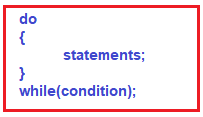##### While and do-while are almost the same. So, what is the difference? Which one do we use?

The difference between the do-while loop and the while loop in C# is that the do-while evaluates its test condition at the bottom of the loop whereas the while loop evaluates its test condition at the top. Therefore, the statements written inside the do-while block are executed at least once whereas we cannot give a guarantee that the statements written inside the while loop are going to be executed at least once.

Note: When you want to execute the loop body at least once irrespective of the condition, then you need to use the do-while loop else you need to use the while loop.

##### Flow Chart of Do-While Loop:

In the do-while loop, first, it will execute the loop body without checking the condition. After executing the loop body, it will check for the condition, and if the condition is true then it will again execute the loop body and if the condition is false then it will terminate the loop. For a better understanding, please have a look at the following diagram which shows the flowchart of the do-while loop.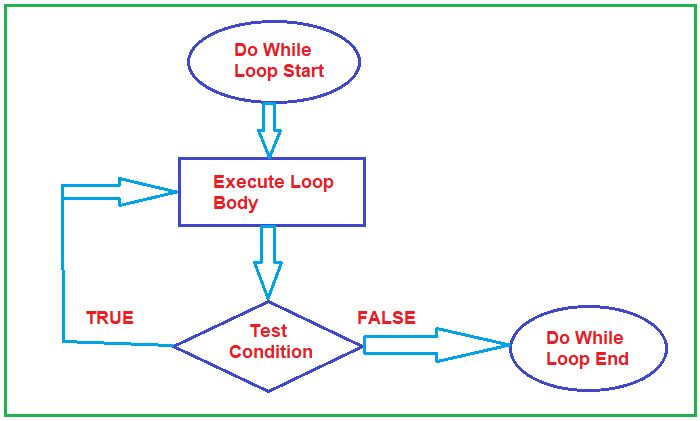##### Example to understand do while loop in C# Language:

In the below example we are printing the numbers from 1 to 5 using the do while loop.

```using System;
namespace ControlFlowDemo
{
class Program
{
static void Main(string[] args)
{
int number = 1;
do
{
Console.Write(\$"{number} ");
number++;
} while (number <= 5);

}
}
}```

Output: 1 2 3 4 5

##### Nested Do-While Loop in C# Language:

Using a do-while loop inside another do-while loop is called nested do-while loop. The syntax to use the nested do-while loop in C# language is given below.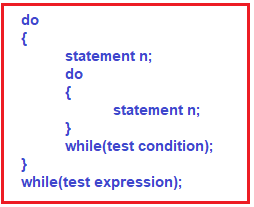##### Example to Understand Nested do-while Loop in C# Language:
```using System;
namespace ControlFlowDemo
{
class Program
{
static void Main(string[] args)
{
do
{
Console.WriteLine("I am from outer do-while loop");
do
{
Console.WriteLine("I am from inner do-while loop ");
}
while (1 > 10);
}
while (2 > 10);

}
}
}
```
###### Output: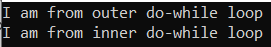##### Realtime Example of Do-While Loop in C#:

Let us understand do while loop with one real-time example. The do-while loop is mainly used in menu-driven programs where the termination condition depends upon the end-user. That means when the end user wants then the loop is going to terminate.

For a better understanding, please have a look at the following example. In the below example, we are using a do while loop, and inside the do while loop we are using a switch case statement. Here, first, we are asking the user to select the options i.e. what operation he wants to perform. The options are 1 for Addition, 2 for Subtraction, 3 for Multiplication, and 4 for Division. Based on the selected options, we are executing the matched case statement and performing the required operations. Once the operation is performed then we are not terminating the loop instead we are asking the user whether he wants to continue or wants to terminate. If he enters the letter y, then again, we are showing the options to select and perform the desired operation and if he enters anything other than y, then the loop will be terminated and will end the program.

```using System;
namespace ControlFlowDemo
{
class Program
{
static void Main(string[] args)
{
char Choice;
int Number1, Number2;
do
{
Console.WriteLine("Press 1 for Addition, 2 for Subtraction, 3 for Multiplication, 4 for Division");
{
case 1:
Console.WriteLine("Enter the value of two numbers");
Console.WriteLine(\$"Sum Is {Number1 + Number2}");
break;
case 2:
Console.WriteLine("Enter the value of two numbers");
Console.WriteLine(\$"Difference Is {Number1 - Number2}");
break;
case 3:
Console.WriteLine("Enter the value of two numbers");
Console.WriteLine(\$"Multiplication Is {Number1 * Number2}");
break;
case 4:
Console.WriteLine("Enter the value of two numbers");
Console.WriteLine(\$"Division Is {Number1 / Number2}");
break;
default:
Console.WriteLine("Invalid choice");
break;
}
Console.WriteLine("Please Enter Y to continue, any keys to terminate");

}
while (Char.ToUpper(Choice) == 'Y');
}
}
}
```
###### Output: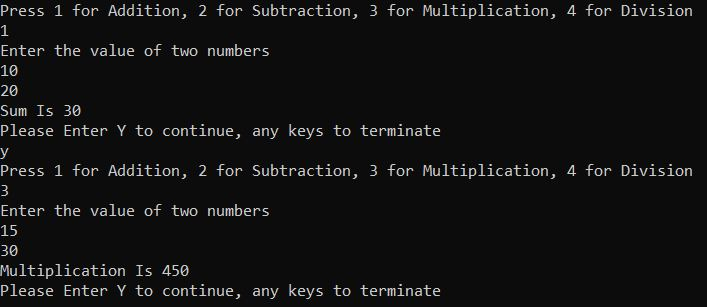1.